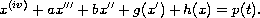Electron. J. Diff. Eqns., Vol. 2006(2006), No. 06, pp. 1-6.

### Boundedness of solutions to fourth order differential equations with oscillatory restoring and forcing terms Babatunde S. Ogundare

Abstract:Using the Cauchy formula for the particular solution of non-homogeneous linear differential equations with constant coefficients, we prove that the solution and its derivatives up to order three are bounded.

Submitted

Submitted September 7, 2005. Published January 11, 2006.
Math Subject Classifications: 34C10, 34C11.
Key Words: Fourth order differential equation; bounded solution; oscillatory solution; restoring and forcing terms.

Show me the PDF file (189K), TEX file, and other files for this article.Babatunde S. Ogundare Department of Mathematics Obafemi Awolowo University, Ile-Ife, Nigeria email: bogunda@oauife.edu.ng   ogundareb@yahoo.com